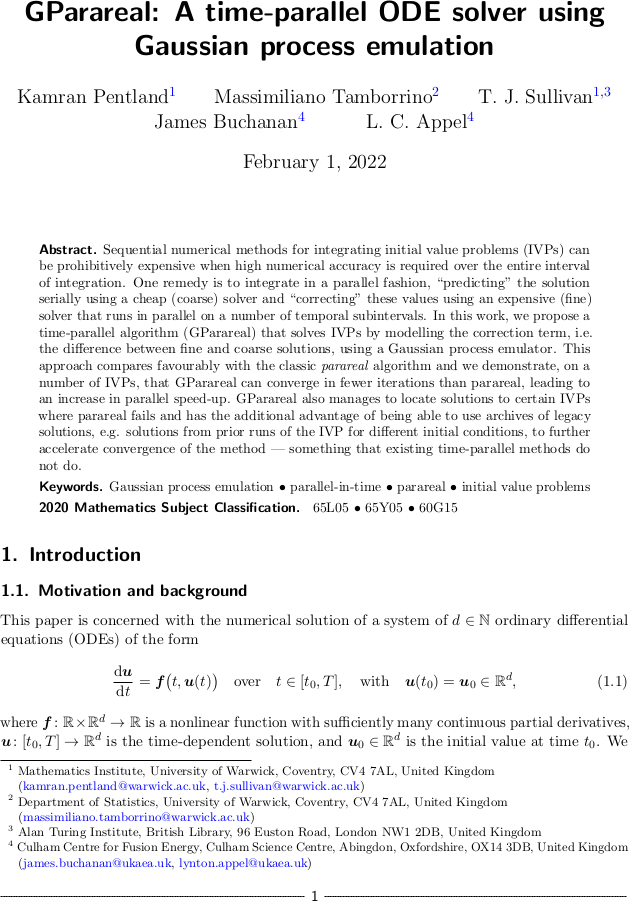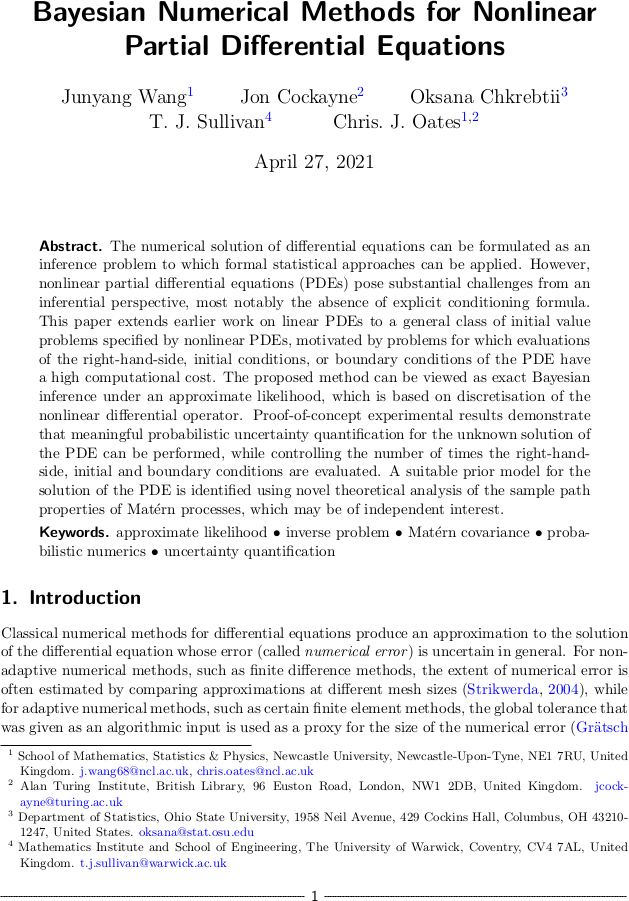# Tim Sullivan

### #prob-num

Clear Search### Randomised integration for deterministic operator differential equations in Calcolo

The article “Randomised one-step time integration methods for deterministic operator differential equations” by Han Cheng Lie, Martin Stahn, and myself has just appeared in its final form in Calcolo. In this paper, we extend the analysis of Conrad et al. (2016) and Lie et al. (2019) to the case of evolutionary systems in Banach spaces or even Gel′fand triples, this being the right setting for many evolutionary partial differential equations.

H. C. Lie, M. Stahn, and T. J. Sullivan. “Randomised one-step time integration methods for deterministic operator differential equations.” Calcolo 59(1):13, 33pp., 2022. doi:10.1007/s10092-022-00457-6

Abstract. Uncertainty quantification plays an important role in applications that involve simulating ensembles of trajectories of dynamical systems. Conrad et al. (Stat. Comput., 2017) proposed randomisation of deterministic time integration methods as a strategy for quantifying uncertainty due to time discretisation. We consider this strategy for systems that are described by deterministic, possibly non-autonomous operator differential equations defined on a Banach space or a Gel′fand triple. We prove pathwise and expected error bounds on the random trajectories, given an assumption on the local truncation error of the underlying deterministic time integration and an assumption that the absolute moments of the random variables decay with the time step. Our analysis shows that the error analysis for differential equations in finite-dimensional Euclidean space carries over to infinite-dimensional settings.

Published on Friday 25 February 2022 at 17:00 UTC #publication #prob-num #lie #stahn### GParareal: A time-parallel ODE solver using Gaussian process emulation

Kamran Pentland, Massimiliano Tamborrino, James Buchanan, Lynton Appel and I have just uploaded a preprint of our latest article, “GParareal: A time-parallel ODE solver using Gaussian process emulation”, to the arXiv. In this paper, we show how a Gaussian process emulator for the difference between coarse/cheap and fine/expensive solvers for a dynamical system can be used to enable rapid and accurate solution of that dynamical system in a way that is parallel in time. This approach extends the now-classical Parareal algorithm in a probabilistic way that allows for efficient use of both runtime and legacy data gathered about the coarse and fine solvers, which may be a critical performance advantage for complex dynamical systems for which the fine solver is too expensive to run in series over the full time domain.

Abstract. Sequential numerical methods for integrating initial value problems (IVPs) can be prohibitively expensive when high numerical accuracy is required over the entire interval of integration. One remedy is to integrate in a parallel fashion, “predicting” the solution serially using a cheap (coarse) solver and “correcting” these values using an expensive (fine) solver that runs in parallel on a number of temporal subintervals. In this work, we propose a time-parallel algorithm (GParareal) that solves IVPs by modelling the correction term, i.e. the difference between fine and coarse solutions, using a Gaussian process emulator. This approach compares favourably with the classic parareal algorithm and we demonstrate, on a number of IVPs, that GParareal can converge in fewer iterations than parareal, leading to an increase in parallel speed-up. GParareal also manages to locate solutions to certain IVPs where parareal fails and has the additional advantage of being able to use archives of legacy solutions, e.g. solutions from prior runs of the IVP for different initial conditions, to further accelerate convergence of the method - something that existing time-parallel methods do not do.

Published on Tuesday 1 February 2022 at 12:00 UTC #preprint #prob-num #pentland #tamborrino #buchanan #appel### Bayesian numerical methods for nonlinear PDEs

Junyang Wang, Jon Cockayne, Oksana Chkrebtii, Chris Oates, and I have just uploaded a preprint of our recent work “Bayesian numerical methods for nonlinear partial differential equations” to the arXiv. This paper continues our study of (approximate) Bayesian probabilistic numerical methods (Cockayne et al., 2019), in this case for the challenging setting of nonlinear PDEs, with the goal of realising a posterior distribution over the solution of the PDE that carries a meaningful expression of uncertainty about the solution's true value given the discretisation error that has been incurred.

Abstract. The numerical solution of differential equations can be formulated as an inference problem to which formal statistical approaches can be applied. However, nonlinear partial differential equations (PDEs) pose substantial challenges from an inferential perspective, most notably the absence of explicit conditioning formula. This paper extends earlier work on linear PDEs to a general class of initial value problems specified by nonlinear PDEs, motivated by problems for which evaluations of the right-hand-side, initial conditions, or boundary conditions of the PDE have a high computational cost. The proposed method can be viewed as exact Bayesian inference under an approximate likelihood, which is based on discretisation of the nonlinear differential operator. Proof-of-concept experimental results demonstrate that meaningful probabilistic uncertainty quantification for the unknown solution of the PDE can be performed, while controlling the number of times the right-hand-side, initial and boundary conditions are evaluated. A suitable prior model for the solution of the PDE is identified using novel theoretical analysis of the sample path properties of Matérn processes, which may be of independent interest.

Published on Tuesday 27 April 2021 at 10:00 UTC #preprint #prob-num #wang #cockayne #chkrebtii #oates### Computing with dense kernel matrices at near-linear cost in MMS

The paper “Compression, inversion, and approximate PCA of dense kernel matrices at near-linear computational complexity” by Florian Schäfer, Houman Owhadi, and myself has just appeared in print in Multiscale Modeling and Simulation. This paper shows how a surprisingly simple algorithm — the zero fill-in incomplete Cholesky factorisation — with respect to a cleverly-chosen sparsity pattern allows for near-linear complexity compression, inversion, and approximate PCA of square matrices of the form

$$\Theta = \begin{bmatrix} G(x_{1}, x_{1}) & \cdots & G(x_{1}, x_{N}) \\ \vdots & \ddots & \vdots \\ G(x_{N}, x_{1}) & \cdots & G(x_{N}, x_{N}) \end{bmatrix} \in \mathbb{R}^{N \times N} ,$$

where $$\{ x_{1}, \dots, x_{N} \} \subset \mathbb{R}^{d}$$ is a data set and $$G \colon \mathbb{R}^{d} \times \mathbb{R}^{d} \to \mathbb{R}$$ is a covariance kernel function. Such matrices play a key role in, for example, Gaussian process regression and RKHS-based machine learning techniques.

F. Schäfer, T. J. Sullivan, and H. Owhadi. “Compression, inversion, and approximate PCA of dense kernel matrices at near-linear computational complexity.” Multiscale Modeling & Simulation: A SIAM Interdisciplinary Journal 19(2):688–730, 2021. doi:10.1137/19M129526X

Abstract. Dense kernel matrices $$\Theta \in \mathbb{R}^{N \times N}$$ obtained from point evaluations of a covariance function $$G$$ at locations $$\{ x_{i} \}_{1 \leq i \leq N}$$ arise in statistics, machine learning, and numerical analysis. For covariance functions that are Green's functions of elliptic boundary value problems and homogeneously-distributed sampling points, we show how to identify a subset $$S \subset \{ 1 , \dots , N \}^2$$, with $$\# S = O ( N \log (N) \log^{d} ( N /\varepsilon ) )$$, such that the zero fill-in incomplete Cholesky factorisation of the sparse matrix $$\Theta_{ij} 1_{( i, j ) \in S}$$ is an $$\varepsilon$$-approximation of $$\Theta$$. This factorisation can provably be obtained in complexity $$O ( N \log( N ) \log^{d}( N /\varepsilon) )$$ in space and $$O ( N \log^{2}( N ) \log^{2d}( N /\varepsilon) )$$ in time; we further present numerical evidence that $$d$$ can be taken to be the intrinsic dimension of the data set rather than that of the ambient space. The algorithm only needs to know the spatial configuration of the $$x_{i}$$ and does not require an analytic representation of $$G$$. Furthermore, this factorization straightforwardly provides an approximate sparse PCA with optimal rate of convergence in the operator norm. Hence, by using only subsampling and the incomplete Cholesky factorization, we obtain, at nearly linear complexity, the compression, inversion and approximate PCA of a large class of covariance matrices. By inverting the order of the Cholesky factorization we also obtain a solver for elliptic PDE with complexity $$O ( N \log^{d}( N /\varepsilon) )$$ in space and $$O ( N \log^{2d}( N /\varepsilon) )$$ in time.

Published on Thursday 15 April 2021 at 12:00 UTC #publication #prob-num #schaefer #owhadi### Randomised integration for deterministic operator differential equations

Han Cheng Lie, Martin Stahn, and I have just uploaded a preprint of our recent work “Randomised one-step time integration methods for deterministic operator differential equations” to the arXiv. In this paper, we extend the analysis of Conrad et al. (2016) and Lie et al. (2019) to the case of evolutionary systems in Banach spaces or even Gel′fand triples, this being the right setting for many evolutionary partial differential equations.

Abstract. Uncertainty quantification plays an important role in applications that involve simulating ensembles of trajectories of dynamical systems. Conrad et al. (Stat. Comput., 2017) proposed randomisation of deterministic time integration methods as a strategy for quantifying uncertainty due to time discretisation. We consider this strategy for systems that are described by deterministic, possibly non-autonomous operator differential equations defined on a Banach space or a Gel′fand triple. We prove pathwise and expected error bounds on the random trajectories, given an assumption on the local truncation error of the underlying deterministic time integration and an assumption that the absolute moments of the random variables decay with the time step. Our analysis shows that the error analysis for differential equations in finite-dimensional Euclidean space carries over to infinite-dimensional settings.

Published on Wednesday 31 March 2021 at 09:00 UTC #preprint #prob-num #lie #stahn